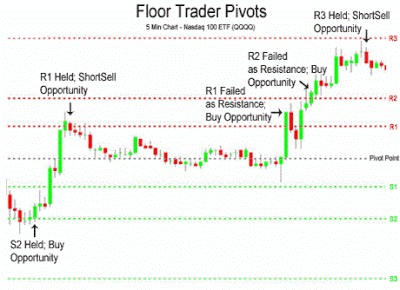# Pivot Points in Trading

The pivot points are a type of market analysis tool used by many investors to trade regularly in any financial market including Forex. They are used in the same way as support and resistance to determine levels at which the price is likely to bounce or continue the current trend. In this article we are going to show the different types of pivot points that exist, their calculation and some suggestions for their use.Pivot Points Calculation

To calculate the pivot points to trade the next market session,  you need the high (H), low (L) and close (close) of the previous session. The pivot point is simply the average of the high plus low plus close, or (H + L + C) / 3. The level of support 1 is calculated by multiplying the Pivot by 2 and then subtracting the previous day’s high. The resistance level 1 is calculated by multiplying the Pivot by 2 and then subtracting the previous day’s low. The secondary support (S2) and the level of resistance (R2) are calculated using the numbers (P, S1 and R1). And finally, the support 3 (S3) and resistance 3 (R3) are calculated using the numbers (P, H, L). The general formulas are the following:

• Resistance 2 (R2) = P + (H – L)
• Resistance 1 (R1) = P + (P – L)
• PP (P) = (H + L + C) / 3
• Support 1 (S1) = PP – (H – P)
• Support 2 (S2) = P – (H – L)
There are variations of the formula, where the support and resistance levels are calculated differently, slightly varying results. Here are some:
• Resistance 2 (R2) = P + (R1 – S1)
• Resistance 1 (R1) = (P * 2) – L
• Pivot Point (P) = (H + L + C) / 3
• Support 1 (S1) = (P * 2) – H
• Support 2 (S2) = P– (R1 – S1)
• Resistance 2 (R2) = P – (S1 + R1)
• Resistance 1 (R1) = (2*P) – L
• PP (P) = (H + L + C) / 3
• Support 1 (S1) = (2*P) – H
• Support 2 (S2) = P – (R1 + S1)
Certain platforms also calculated levels (S3) and (R3), useful in the days of high volatility, while other can calculate intermediate levels, called (M), which also provide additional information. These levels can be are useful after a day with high volatility, as the pivot points are very far between them.
• Resistance 3 (R3) = H + 2 X (P – L)
• Resistance2 (R2) = P + H – L
• Resistance 1 (R1) = (2 X P) – L
• PP (P) = (H + L + C) / 3
• Support 1 (S1) = (2 X P) – H
• Support 2 (S2) = P – H + L
• Support 3 (S3) = L – 2 X (H – P)

## Variants of the Pivot Points

### Tom Demark Pivot Points

Another popular method for calculating pivot points that can be used to predict the future of a market trend are the pivot points of Tom Demark, which in reality are not exactly pivot points, but are used to calculate the maximum and minimum of a period. To calculate these levels we can use following formulas:
• If Close < Open, then X = H+2×L+C
• If Close > Open, then X = 2xH+L+C
• If Close = Open, then X = H+L+2xC
• New Maximum = X / 2 – L
• New Minimum = X / 2 – H

### Camarilla Pivot Points

Camarilla Pivot Points are a set of 8 levels that have a high probability of behaving like support and resistance for the current trend. According to traders operating on the basis of these levels are quite effective to place stop loss orders (to limit losses) and take profit orders (for profit-taking) for which its use has been popularized in recent years. To calculate these pivot points are used the following formula:
• R4 = (H – L) X 1.1 / 2 + C
• R3 = (H – L) X 1.1 / 4 + C
• R2 = (H – L) X 1.1 / 6 + C
• R1 = (H – L) X 1.1 / 12 + C
• S1 = C – (H – L) X 1.1 / 12
• S2 = C – (H – L) X 1.1 / 6
• S3 = C – (H – L) X 1.1 / 4
• S4 = C – (H – L) X 1.1 / 2
More information on the Camarilla pivot points in the following article: